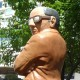# Clipping Images in PDFs: Now With More Symmetry

I’ve recently become a bit obsessed with symmetry about a point. As demonstrated with PDF Images of course. In theory, it should be simple: just rotate an image about a point.

## Iteration 0: Rotate the Image.

So let’s see the result:## Iteration 1: Remove the Overlap

There is the start of a hint of symmetry there. But it is spoiled when the last copy of the image is positioned on the page. Let’s spread out the images so that they don’t overlap:Okay, that’s symmetrical. And notice that the trapped white space inside forms a convex regular polygon. Since we know the length of each side is the width of the image, we can calculate a circumradius. We can then position each image a circumradius away from the center and rotate the image to be tangent to the (implied) circle. So here is what changed:

Rather than starting from arbitrary angle 0, we go around the unit circle starting from an arbitrary start angle parameter specified in degrees.

We extract the image width and calculate the circumradius. And for the rotation of the images, we calculate this rotation angle constant:

The translation matrix is now a bit more complicated:

Likewise, the rotation:

Now let’s try bringing that back in to the center point.  If you look at the image above, to bring it back to the center you need to move each image by a vector. The magnitude is essentially the apothem of the regular polygon. And the direction is essentially the opposite of the rotation angle.  Translated into code:

The rotation angle is split into two: a pure rotation angle, and a rotation angle for the image itself.

Which changes the translation matrix to:

and the result is below.Not bad, but we still have the same issue we started with, the overlapping.

## Iteration 3: Add in Clipping

To achieve better symmetry, we are going to need to reduce the image overlap, which means that we are going to need to clip the image, and rotate the clip along with the image. Now the clip is either going to be 5-sided, or 3-sided. With a portrait-oriented picture like above, it’s going to be 5-sided, because the cuts are going to hit the left and right sides of the image, and then go straight up and across. But with a wider image, the cuts might go through the top edge of the image, and the result will be a triangle. For this calculation, we will need to know the height of the image.

Then we determine the shape and the vertices of that shape:

From which we create a clip path:

Which we will clone and add to each image clone after translating and rotating it to match the image clone.

For a final result of:It looks pretty (symmetrical) to me!
Here’s another statue rotated 8 times around a point:Note that a triangular clipping path is used for this image.

## Iteration 4: More Clipping

Now, what if we don’t want the bottom of the image to be exactly at the center of the point? What if we want to push it back out a bit, by an offset?
That changes the translation matrix slightly with the offset worked in:

But the action is really with the shape of the clip which now has an extra side added to it.The final iteration of this code is significantly more complicated than its first, naive, iteration, but it followed a generally logical progression. There are at least a couple of different ways we could continue extending this code, but I’m going to leave that for a future blog post.